# Kerala Syllabus 9th Standard Chemistry Solutions Chapter 2 Chemical Bonding

You can Download Chemical Bonding Questions and Answers, Summary, Activity, Notes, Kerala Syllabus 9th Standard Chemistry Solutions Part 1 Chapter 2 help you to revise complete Syllabus and score more marks in your examinations.

## Kerala State Syllabus 9th Standard Chemistry Solutions Chapter 2 Chemical Bonding

### Chemical Bonding Textual Questions and Answers

Kerala Syllabus 9th Standard Chemistry Notes Chapter 2 Question 1.
What peculiarity do you see in the electronic configuration of noble elements except Helium? Except Helium all other elements have 8 electrons in the outermost shell, hence shall be considered to be chemically stable.

The arrangement of eight electrons in the outermost shell of atom is called octet electron configuration. In Helium atom there is only one shell. The maximum number of electrons in the first shell is 2. Hence two-electron pattern system of Helium also stable.

Kerala Syllabus 9th Standard Chemistry Chapter 2 Question 2.
The electronic configuration of some elements are given below.

 Element Atomic mass Electronic configuration Magnesium 12 2, 8, 2 Oxygen 8 2, 6 Sodium 11 2, 8, 1 Chlorine 17 2, 8, 7

Is the number of electrons in the outermost shell of these elements the same as that of the elements in Table (2.1).
No

(i) You are familiar with the compounds of these elements. Write the names of some compounds?
Magnesium chloride, Sodium oxide, Sodium chloride.

(ii) How are atoms in these compounds held together?
Strong attractive force

(iii) What is meant by Chemical Bonding?
The attractive force that holds the atoms together in the formation of a molecule is called chemical bonding.

Ionic Bonding

Class 9 Chemistry Chapter 2 Notes Kerala Syllabus Question 3.
In the formation of sodium chloride which atoms are combing.
Sodium, chlorine

Chemical Bonding Notes Class 9 Kerala Syllabus Question 4.
How many electrons are there in the outermost shell of sodium atom?
1

Chemical Bonding Questions And Answers Class 9 Kerala Syllabus Question 5.
How many electrons are there in outermost shell of chlorine?
7

Chemical Bonding Questions And Answers Pdf Class 9 Kerala Syllabus Question 6.
How do chlorine and sodium attain stability?
Sodium donates one electron to chlorine to become sodium ion [Na+] and chlorine become chloride ion [Cl]

Chemical Bonding Class 9 Notes Pdf Kerala Syllabus Question 7.
Analyze the electron transfer in each atom during the formation of sodium chloride.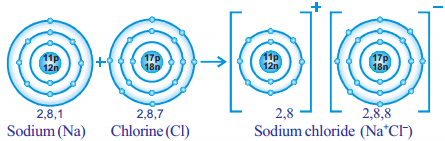Chemical Bonding Class 9 Pdf Kerala Syllabus Question 8.
Draw the electron dot diagram of the transference of electron of sodium atom and chlorine atom. The diagram represents only electrons in the outermost shell because they are the only electrons participating- in chemical bonding.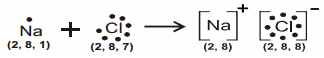Questions On Chemical Bonding Class 9 Kerala Syllabus Question 9.
Complete Table 2.3 by examining the arrangement of electrons before and after the chemical reaction during the formation of sodium chloride.a) Which atom donates electron? How many electrons?
b) Which atom accepts electron? How many electrons?
a) Sodium, one electron
b) Chlorine, one electron

Kerala Syllabus 9th Standard Chemistry Notes English Medium Question 10.
Electron transfer during the formation of sodium chloride can be written in the form of an equation
Na → Na++1e
Cl + 1e → Cl
During the formation of sodium chloride sodium atom donates electron and gets converted to sodium ion (Na+) chlorine accepts an electron to form chloride ion (Cl ). Through this sodium and chlorine atoms complete an octet in their outermost shell to attain stability.

The oppositely charged ions thus formed are held together by electrostatic force of attraction. This attractive force is called Ionic Bond. Sodium chloride contains ionic bond.

Kerala Syllabus 9th Standard Chemistry Chapter 1 Question 11.
Define Ionic Bond?
Ionic bond is a chemical bond formed by electron transfer in an ionic bond, the ions are held together by the electrostatic force of attraction between the oppositely charged ions.

Kerala Syllabus 9th Standard Chemistry Solutions Question 12.
Explain the formation of magnesium oxide from magnesium and oxygen?
Analyze the electron dot diagram and complete the table.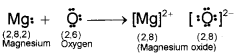To attain stability magnesium donates 2 electrons to become magnesium ion (Mg2+) and – oxygen become [O2 ] ion. This type of bonding is ionic bonding.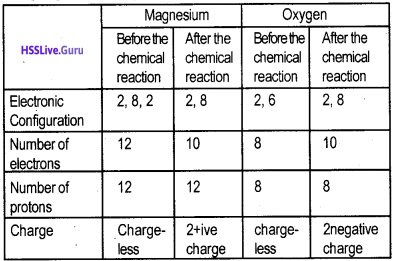Chemical Bonding Notes Class 9 Pdf Kerala Syllabus Question 13.
How the ionic bond formation of sodium oxide is represented?
[Hint: Atomic No. of sodium 11, oxygen 8]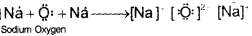Question 14.
Draw the electron dot diagram of following compounds. [Hint: Atomic No. Na=11, F=9, Mg=12]
1. Sodium Flouride [NaF]Question 15.
Define ionic compounds?
Compounds formed by ionic bonding are called ionic compound.

Covalent Bonding

Fluorine [F2], Chlorine [Cl2] oxygen [O2] Nitrogen [N2] etc. are diatomic molecules. Let us examine the formation of these molecules.
The Bohr atom model of fluorine is given in figure.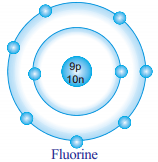Question 16.
Write the atomic number of fluorine?
9

Question 17.
The electronic configuration of Fluorine
2, -7 ‘

Question 18.
How many electrons are required for one fluorine atom to attain the octet?
1

Question 19.
Is there a possibility of transferring electrons from one fluorine atom to another fluorine atom?
No.

Question 20.
How can the two fluorine atoms attain an octet arrangement?
By sharing of electrons

Question 21.
The manner in which the two fluorine atoms in a fluorine molecule undergo chemical bonding is illustrated in fig. 2.6.Question 22.
What happens during the formation of fluorine molecule electron transfer or electron sharing?
Electron sharing

Question 23.
How many pairs of electrons are shared?
One pair

Question 24.
How covalent bonds are formed?
The chemical bond formed as a result of the sharing of electrons between the combining atoms is called a covalent bond.

Question 25.
How single bonds are formed?
Single bonds are formed by sharing one pair of electrons. It is represented by a small line between the symbols of the combining element, eg. Fluorine molecules can be represented as F – F. The atomic number of chlorine is 17.

Question 26.
Write down the electronic configuration?
2, 8, 7

Question 27.
Draw the electron dot diagram of the formation of chlorine molecule by combining two chlorine atoms?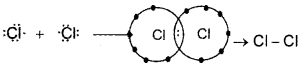Here one pair of electrons – sharing hence single bond is formed.

Question 28.
Examine the diagram illustrating the chemical bonding in the molecule of oxygen and nitrogen.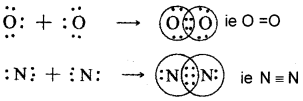In oxygen molecules, two pairs of electrons are shared hence this type of covalent bond is double bond.
In nitrogen molecule, three pairs of electrons are shared hence this type of covalent bond is triple bond.

Question 29.
Complete table 2.5 given below related to covalent bonding.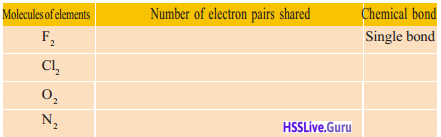Element molecule Shared electron pairs Chemical bond F2 One pair Single bond Cl2 One pair Single bond O2 Two pair Double bond N2 Three pairs Triple bond

Question 30.
Draw the chemical bond formation of hydrogen chloride [HCI]a) How many electron pairs are shared?
b) Represent chemical bond by using symbols?
a) One pair of electrons
b) H – Cl

Question 31.
Examples of some covalent compounds are given draw the chemical bonds of the compound by using electron dot diagram.
a)CH4
b) HF
c)H2O
a)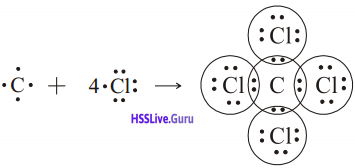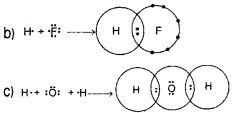Electronegativity

Question 32.
Define electronegativity?
In a covalent bond the relative ability of each atom to attract the bonded pair of electrons towards itself is called electronegativity.

Question 33.
Who proposed the electronegativity scale?
Linus Pauling

Question 34.
Some compounds and their nature are shown in table (2.6) complete the table by finding out the electron negativity difference between the constituent elements.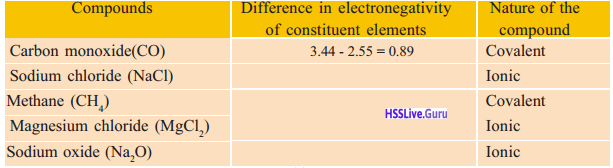Compounds Electronegativity difference of the elements Character of compound Carbon Tetra Chloride [CCl4] 3.44 – 2.55 = 0.89 Covalent bond Sodium chloride [NaCl] 3.16 – 0.98 = 2.23 Ionic bond Methane [CH4] 2.55 – 2.20 = 0.35 Covalent bond Magnesium chloride [MgCl2] 3.16 – 1.31 = 1.85 Ionic bond Sodium oxide[Na2O] 3.44 – 0.93 = 2.51 Ionic bond

Generally, the electronegativity difference of the component elements in a compound is 1.7 or more it shows ionic character. If it is less than 1.7 it shows covalent character.

Polar Nature

Question 35.
Consider the case of hydrogen molecule [HCl]
a) What is the electronegativity of hydrogen?
b) What is the electronegativity of chlorine?
c) The atomic nucleus of which of these elements has a greater tendency to attract the shared pair of electrons?
d) The chlorine atom with a higher electronegativity attracts the shared pair of electrons towards its nucleus. As a result, the chlorine atom in hydrogen chloride develops a partial negative charge g: (delta negative) and hydrogen atom develops a partial positive charge δ+ (delta positive) it can be represented below
a) 2.2
b) 3.16
c) Chlorine
d) $$\begin{array}{l}{\delta^{+} \quad \quad \delta^{-}} \\ {H-C^{\prime}}\end{array}$$ Compounds having partial electron charge separation with the molecule are called polar compound. HF, HBr, H20 are example of polar compounds.

Question 36.
Explain the properties of Ionic compounds and covalent compounds.

 Properties Ionic compound Covalent compounds State Solid Found in the three states solids, liquids and gases Solubility in water soluble in water Insoluble in water. But soluble in organic solvent like kerosene, benzene etc. Electrical Conductivity conduct electri­city in fused or solution state Do not conduct Electricity Melting point Boiling point High Generally Low

Valency:

Question 37.
What is meant by valency?
Valency is the combining capacity of the atoms of an element. It can be treated as the number of electrons lost gained or shared by an atom during chemical combination.

Question 38.
In the formation of sodium chloride- sodium donates one electron, chlorine accepts one electron write the valencies of each element?
1

Question 39.
In the formation of magnesium oxide- How many electrons are donated by magnesium?
2

Question 40.
How many electrons are accepted by oxygen?
2

Question 41.
How is valency and electron transfer related in this case?
Same

Question 42.
In the formation of hydrogen chloride, how many electron pairs are shared?
One pair

Question 43.
What will be the valency of each atom?
1

Question 44.
Complete the table given below analyze the change in the electronic arrangement of elements during the formation of each compound. Find how they are related to valency.Compound Component elements Atomic number Electron configur­ation No. of elect­rons donated accepted/ shared Valency NaCl Na 11 2, 8, 1 1 1 Cl 17 2, 8 ,7 1 1 MgO Mg 12 2, 8, 2 2 2 0 8 2, 6 2 2 HF H 1 1 1 1 F 9 2, 7 1 1 CCl4 C 6 2, 4 4 4 Cl 17 2, 8, 7 1 1

From Valency to Chemical Formula

The chemical formula of some compounds are given
Sodium chloride – NaCl
Magnesium chloride – MgCl2
Aluminium chloride – AICl3
Carbon tetrachloride – CCl4

Question 45.
The symbol of some elements and there valencies are given. Write the chemical formula of the compounds formed by them.

 Element Valency Cl 1 Li 1 0 2 Zn 2 Ca 2Question 46.
Why does the number of chlorine atoms differ in these compounds? Try to find out by analyzing the valency of the elements Na, Mg, Al, Cl and C. Analyse Table 2.9

Examine the above table and identify how to write the chemical formula from valency. Compare your findings with the following.

• First write the element with lower electronegativity.
• Exchange the valency of each element and write as suffix.
• Divide the suffix with the common factor.
• If the suffix is 1, it need not be written.

Let Us Assess

Question 1.
Complete the table given below and answer the following questions (symbols used are not true)

 Element Atomic number Electronic configuration P 9 2, 7 Q 17 ………………………….. R 10 ………………………….. S 12 ……………………………

a) Which element in the table is the most stable one? Justify your answer.
b) Which element donates electrons in chemical reaction?
c) Write the chemical formula of the compound formed by combining element S with P.

 Element Atomic number Electronic configuration P 9 2, 7 Q 17 2, 8, 7 R 10 2, 8 S 12 2, 8, 2

a) R – it contains an octet configuration in the outermost shell.
b) S
c) The valency of S = 2 and P is 1, hence the chemical formula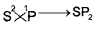[The element in which the electron lose can be written first]

Question 2.
Electronegativity values of some elements are given. Using these values, find whether the following compounds are ionic or covalent.
(Electronegativity of Ca = 1, O = 3.5, C = 2.5, S = 2.58, H = 2.2, F = 3.98)
i) Sulphur dioxide (S02)
ii) Water (H2O)
iii) Calcium fluoride (CaF2)
iv) Carbon dioxide (CO2)
i) SO2
Electronegativity difference = 3.5 – 2.58 = 0.92
If the electronegativity difference is less than 1.7, it shows covalent character.
ii) Water (H2O)
Electronegativity difference = 3.5 – 2.2 = 1.3
If the electronegativity difference is less than 1.7 it forms covalent compounds.
iii) CaF2
Electronegativity difference = 3.98 -1.0 = 2.98
If the electronegativity difference is greater than 1.7 if forms ionic compounds.
iv) CO2
Electronegativity difference = 3.5 – 2. 5 = 1.0
If the electronegativity difference is less than 1.7 it shows covalent compounds.

Question 3.
Some elements and their valencies are given

 Element Valency Ba 2 Cl 1 Zn 2 O 2

a) Write the chemical formula of barium chloride
b) Write the chemical formula of zinc oxide
c) The chemical formula of calcium oxide is CaO. What is the valency of calcium?c) The valency of calcium is 2.

Question 4.
Examine the following chemical equations and answer the questions.
(Hint: Atomic Number Mg = 12 Cl = 17)
Mg + Cl2 → MgCl2
Mg → Mg2+ + ……..
Cl + 1e → ………..
a) Complete the chemical equations.
b) Which is the cation? Which is the anion?
c) Which type of chemical bond is present in MgCl2?
a) Mg → Mg2- + 2e
Cl + 1e → Cl
b) The cation or positively charged ion is Mg2+ and the anion or negatively charged ion is Cl
c) Ionic Bonding

Extended Activities

Question 1.
Draw the electron dot diagram of chemical bonds in methane (CH4) and ethane (C2H6).Question 2.
P, Q, R, S are four elements. Their atomic numbers are 8, 17, 12 and 16 respectively. Find the type of chemical bond in these compounds formed by combining the following pairs of elements. Construct and exhibit the type of bonds using different. substances (eg. pearls) (Electronegativity values:
P = 3.44, Q = 3.16, R = 1.31, S = 2.5)
1) P, R
2) P, S
3) Q, R
1. PR
Electronegativity difference = 3.44 – 1.31 = 2.13
The electronegativity difference is greater 1.7 it shows ionic compound.
2. PS
Electronegativity difference = 3.44 – 2.58 = 0.86
The electronegativity difference is less than 1.7 it shows covalent compound.
3. QR
The electronegativity difference = 3.16 – 1.31 = 1.85
The electronegativity difference is greater than 1.7 it shows ionic compound.

Question 3.
Perform the experiments arranging the apparatus as shown in figure.Record your observations and identify what type of compounds sodium chloride and sugar are